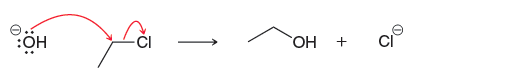# Problem: Consider the following reaction: This reaction has been determined to be second order.How will the rate be affected if the concentration of hydroxide is tripled?

97% (55 ratings)
###### Problem Details

Consider the following reaction: This reaction has been determined to be second order.

How will the rate be affected if the concentration of hydroxide is tripled?Frequently Asked Questions

What scientific concept do you need to know in order to solve this problem?

Our tutors have indicated that to solve this problem you will need to apply the Rate Calculations concept. If you need more Rate Calculations practice, you can also practice Rate Calculations practice problems.

What is the difficulty of this problem?

Our tutors rated the difficulty ofConsider the following reaction: This reaction has been dete...as medium difficulty.

What textbook is this problem found in?

Our data indicates that this problem or a close variation was asked in Organic Chemistry - Klein 1st Edition. You can also practice Organic Chemistry - Klein 1st Edition practice problems.